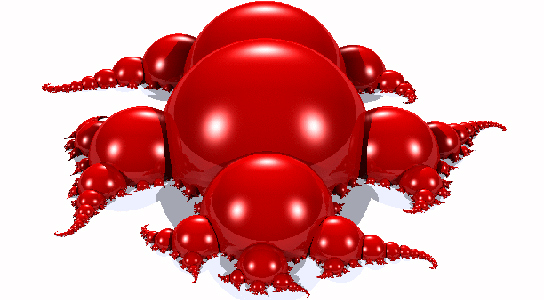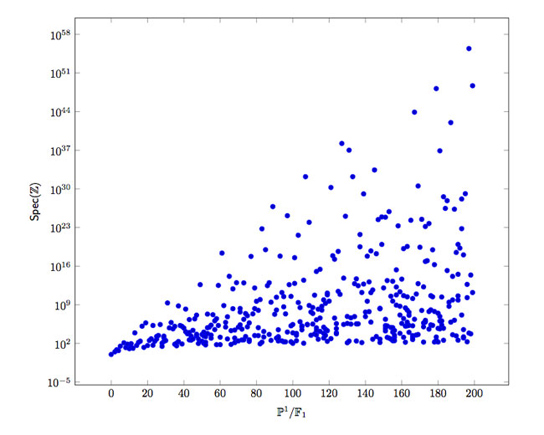# New Mathematical Proof of the ABC ConjectureA pleated surface on the boundary of the convex core.

A new claim could imply that a proof of one of the most important conjectures in number theory has been solved, which would be an astounding achievement. Mathematician Shinichi Mochizuki of Kyoto University in Japan has released a 500-page proof of the abc conjecture that proposes a relationship between whole numbers (related to the Diophantine equations).

The abc conjecture was first proposed by David Masser in 1988 and Joseph Oesterle in 1985. It’s an integer analog to the Mason–Stothers theorem for polynomials. It states that a, b, and c, having no common factors and satisfying a + b = c. If d denotes the product of the distinct prime factors of abc, the conjecture states that d is rarely much smaller than c.If proven true, the abc conjecture could with one stroke solve many famous Diophantine problems, including Fermat’s Last Theorem (which states that an+bn=cn has no integer solutions if n>2). Like many Diophantine problems, it’s about the relationship between prime numbers. It basically encodes a deep connection between the prime factors of a, b, and a+b.

Previous attempts have proven to be flawed. Mochizuki attacked the problem using the theory of elliptic curves, generated by the algebraic relationships of y2=x3+ax+b. From there on, Mochizuki developed techniques that are harder to comprehend, invoking new mathematical constructs and objects, analogous to geometric objects, sets, permutations, topologies, and matrices. He refers to this as inter-universal Teichmüller theory, which generalizes the foundations of algebraic geometry in terms of schemes first envisioned by Grothendieck.

If proven correct, these techniques could provide powerful insights into solving future problems in number theory.